Tamilnadu State Board New Syllabus Samacheer Kalvi 12th Maths Guide Pdf Chapter 12 Discrete Mathematics Ex 12.1 Textbook Questions and Answers, Notes.

## Tamilnadu Samacheer Kalvi 12th Maths Solutions Chapter 12 Discrete Mathematics Ex 12.1

Question 1.
Determine whether * is a binary operation on the sets-given below
(i) a * b – a. |b| on R
(ii) a * b = min (a, b) on A = {1, 2, 3, 4, 5}
(iii) (a * b) = a√b is binary on R
Solution:
(i) Yes.
Reason: a, b ∈ R. So, |b| ∈ R when b ∈ R
Now multiplication is binary on R
So a|b| ∈ R when a, b ∈ R.
(Le.) a * b ∈ R.
* is a binary operation on R.

(ii) Yes.
Reason: a, b ∈ R and minimum of (a, b) is either a or b but a, b ∈ R.
So, min (a, b) ∈ R.
(Le.) a * b ∈ R.
* is a binary operation on R.

(iii) a* b = $$a \sqrt{b}$$ where a, b ∈ R.
No. * is not a binary operation on R.
Reason: a, b ∈ R.
⇒ b can be -ve number also and the square root of a negative number is not real.
So $$\sqrt{b}$$ ∉ R even when b ∈ R.
So $$\sqrt{b}$$ ∉ R. ie., a * b ∉ R.
* is not a binary operation on R.Question 2.
On Z, define ⊗ by (m ⊗ n) =mⁿ + nm: ∀m, n ∈ Z Is ⊗ binary on Z?
Solution:
No. * is not a binary operation on Z.
Reason: Since m, n ∈ Z.
So, m, n can be negative also.
Now, if n is negative (Le.) say n = -k where k is +ve.Similarly, when m is negative then nm ∉ Z.
∴ m * n ∉ Z. ⇒ * is not a binary operation on Z.

Question 3.
Let * be defined on R by (a * b) = a + b + ab – 7. Is * binary on R? If so, find 3 * ($$\frac { -7 }{ 15}$$)
Solution:
(a * b) = a + b + ab – 7 ∀ a, b ∈ R
If a ∈ R, b ∈ R then ab ∈ R
∴ (a * b) = a + b + ab – 7 ∈ R
For example, let 1, 2 ∈ R
(1 * 2) = 1 + 2 + (1)(2) – 7
= -2 ∈ R
∴ * is a binary operation on R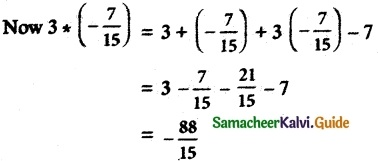Question 4.
Let A = {a + √5 b: a, b ∈ Z}. Check whether the usual multiplication is a binary operation on A.
Solution:
Let A = a + $$\sqrt{5}$$ b and B = c + $$\sqrt{5}$$d, where a, b, c, d ∈ M.
Now A * B ={a + $$\sqrt{5}$$b)(c + $$\sqrt{5}$$d)
= ac + $$\sqrt{5}$$ad + $$\sqrt{5}$$bc + $$\sqrt{5}$$b$$\sqrt{5}$$d
= (ac + 5bd) + $$\sqrt{5}$$(ad+ bc) ∈ A
Where a, b, c, d ∈ Z
So * is a binary operation.

Question 5.
(i) Define an operation * on Q as follows:
a * b = ($$\frac { a+b }{ 2}$$); a, b ∈ Q. Examine the closure, commutative and associate properties satisfied by * on Q.
(ii) Define an operation * on Q as follows: a * b = ($$\frac { a+b }{ 2}$$); a, b ∈ Q. Examine the existence of identity and the existence of inverse for the operation * on Q.
Solution: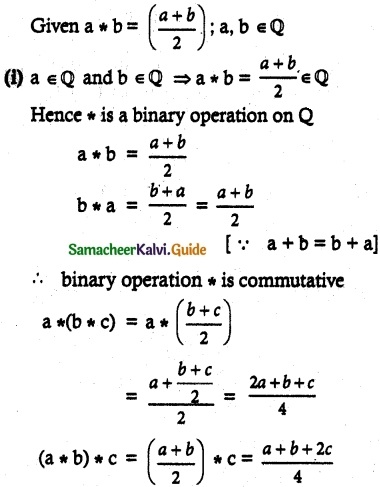so, a * (b * c) ≠ (a * b) * c
Hence, the binary operation * is not associative.(ii) a * b = ($$\frac { a+b }{ 2}$$); a, b ∈ Q
For identity, a * e = e * a = a
Now; a * e = a
$$\frac { a+e }{ 2}$$ = a
a + e = 2a
e = 2a – a = a
Which is not possible
∴ Identity does not exist and hence the inverse does not exist.

Question 6.
Fill in the following table so that the binary operation * on A = {a, b, c} is commutative.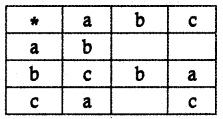Solution:
Given * is commutative on A = {a, b, c}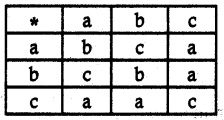From the table, it is given that b * a = c
⇒ a * b = c
as * is commutative
c * a = a = a * c and
b * c = a = c * bQuestion 7.
Consider the binary operation * defined on the set A = {a, b, c, d} by the following table: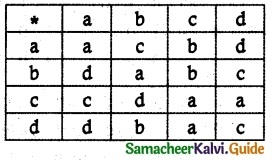Solution:
* is defined on the set A = {a, b, c, d} Given table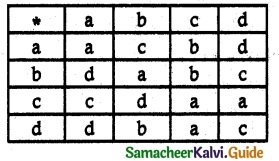From the table a * b = c and b * a = d
⇒ a * b ≠ b * a
∴ * is not commutative.
Now(a * b) * c = c * c = a
a * (b * c) = a * b = c
⇒ (a * b) * c ≠ a * (b * c)
∴ * is not associative

Question 8.
Let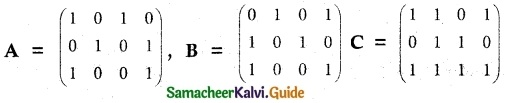be any three boolean matrices of the same type. Find
(i) A v B
(ii) A ∧ B
(iii) (A v B) ∧ C
(iv) (A ∧ B) v C.
Solution:
Given boolean matrices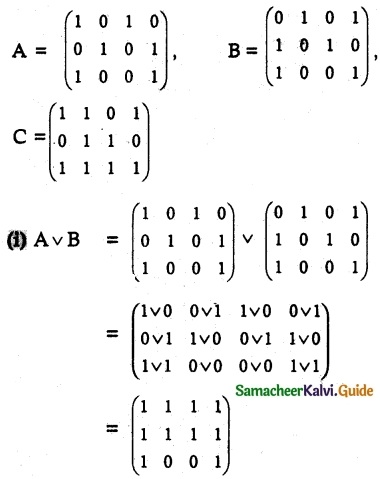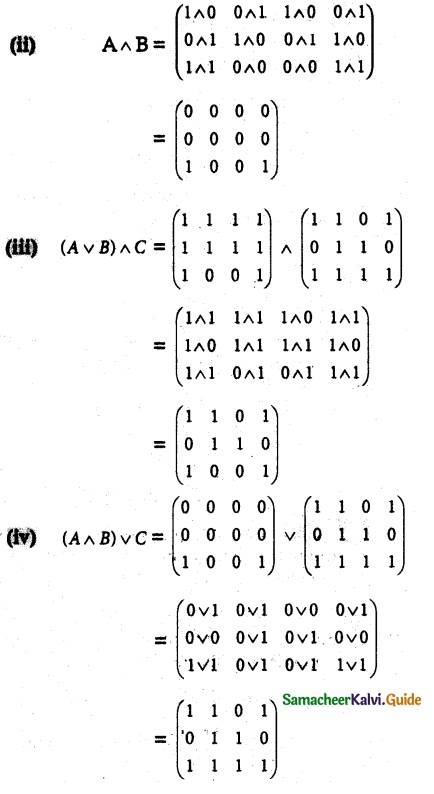Question 9.
(i) Let M = $$\left\{\left(\begin{array}{ll} x & x \\ x & x \end{array}\right): x \in R-\{0\}\right\}$$ and let * be the matrix multiplication. Determine whether M is closed under *. If so, examine the commutative and associative properties satisfied by * on M.
(ii) Let M = $$\left\{\left(\begin{array}{ll} x & x \\ x & x \end{array}\right): x \in R-\{0\}\right\}$$ and let * be the matrix multiplication. Determine whether M is closed under *. If so, examine the existence of identity, existence of inverse properties for the operation * on M
Solution: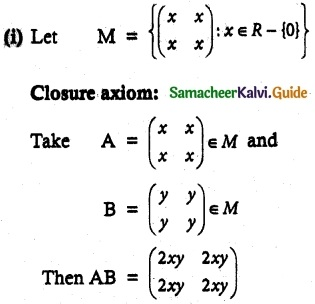Since x ≠ 0, y ≠ 0, we see that 2xy ≠ 0 and so AB ∈ M. This shows that M is closed under matrix multiplication.
Commutative axiom:
AB = $$\left(\begin{array}{ll} 2xy & 2xy \\ 2xy & 2xy \end{array}\right)$$
= BA for all A, B ∈ M
Here, Matrix multiplication is commutative (though in general, matrix multiplication is not commutative)
Associative axiom:
Since matrix multiplication is associative, this axiom holds goods for M.

(ii) Identity axiom: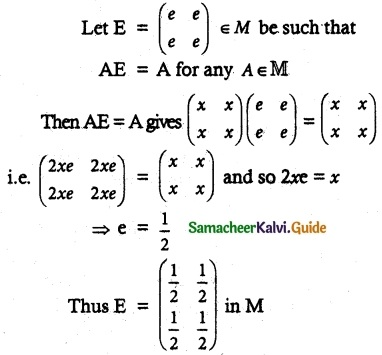Also, we can show that EA = A
Hence E is the identity element in M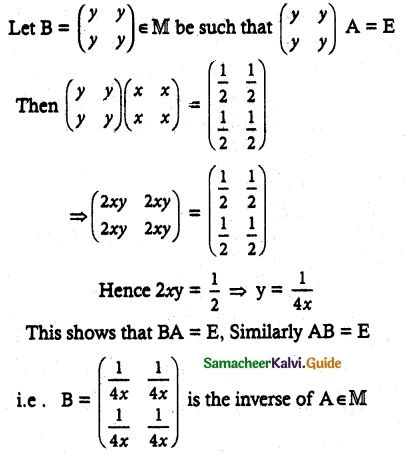Question 10.
(i) Let A be Q\{1} Define * on A by x * y = x + y – xy. Is * binary on A ? If so, examine the commutative and associative properties satisfied by * on A.
(ii) Let A be Q\{1}. Define * on A by x * y = x + y – xy. Is * binary on A ? If so, examine the existence of an identity, the existence of inverse properties for the operation * on A.
Solution:
(i) Let a, b ∈ A (i.e.) a ≠ ±1 , b ≠ 1
Now a * b = a + b – ab
If a + b – ab = 1 ⇒ a + b – ab – 1 = 0
(i.e.) a(1 – b) – 1(1 – b) = 0
(a – 1)(1 – b) = 0 ⇒ a = 1, b = 1
But a ≠ 1 , b ≠ 1
So (a – 1) (1 – 6) ≠ 1
(i.e.) a * b ∈ A. So * is a binary on A.

To verify the commutative property:

Let a, b ∈ A (i.e.) a ≠ 1 , b ≠ 1
Now a * b = a + b – ab
and b * a = b + a – ba
So a * b = b * a ⇒ * is commutative on A.

To verify the associative property:
Let a, b, c ∈ A (i.e.) a, b, c ≠ 1
To prove the associative property we have to prove that
a * (b * c) = (a * b) * c

LHS: b * c = b + c – bc = D(say)
So a * (b * c) = a * D = a + D – aD
= a + (b + c – bc) – a(b + c – bc)
= a + b + c – bc – ab – ac + abc
= a + b + c – ab – bc – ac + abc …… (1)

RHS: (a * b) = a + b – ab = K(say)
So (a * b) * c = K * c = K + c – Kc
= (a + b – ab) + c – (a + b – ab) c
= a + b – ab + c – ac – bc + abc
= a + b + c – ab – bc – ac + abc ….. (2)

(ii) To verify the identity property:
Let a ∈ A (a ≠ 1)
If possible let e ∈ A such that
a * e = e * a = a
To find e:
a * e = a
(i.e.) a + e – ae = aSo, e = (≠ 1) ∈ A
(i.e.) Identity property is verified.
To verify the inverse property:
Let a ∈ A (i.e. a ≠ 1)
If possible let a’ ∈ A such that
To find a’:
a * a’ = e
(i.e.) a + a’ – aa’ = 0
⇒ a'(1 – a) = – a⇒ For every ∈ A there is an inverse a’ ∈ A such that
a* a’ = a’ * a = e
⇒ Inverse property is verified.